# K-means from scratch in R

K-means is an unsupervised machine learning clustering algorithm. It can be used to cluster a set of observations based on similarity between the observations. K-means is one of the most popular clustering technique and it is quite simple to understand.

## K-means clustering algorithm

The goal of this algorithm is to the find the optimal division of n observations into k clusters, so that the total squared distance of the group members to the cluster centroid is minimized.

$W(C_k) =\Sigma_{x_i\in C_k}(x_i-\mu_k)^2$
• $x_i$ is an observation assigned to cluster $C_k$
• $\mu_k$ is the mean value of all observations assigned to cluster $C_k$ (i.e, the centroid)

The K-means algorithm attempts to do the follow:

1. Initialize k number of clusters.
2. k number of observations are randomly selected to be the initial centroids.
3. Determine the distance between observations and the centroids. This can be done by a variety of distance metrics. A common one is the Euclidean distance.
4. Assign each observation to the nearest centroid.
5. Recalculate new centroid position. This is done by updating the centroid coordinates by taking the average of all values of each observations that are part of the cluster.
6. Steps 3 - 5 are repeated iteratively until a maximum number of iterations are reached or the observations no longer assigned to another cluster.

## Computing k-means Clustering

We can develop a simple K-means function using the above algorithm. Here we have a data set USArrests, which contains statistics for arrests per 100,000 residents in each state for either murder, assault, or rape. In addition, the percentage of people living in urban areas is also listed.

library(ggplot2)
library(dplyr)

##
## Attaching package: 'dplyr'

## The following objects are masked from 'package:stats':
##
##     filter, lag

## The following objects are masked from 'package:base':
##
##     intersect, setdiff, setequal, union

library(tidyr)

data("USArrests")

##            Murder Assault UrbanPop Rape
## Alabama      13.2     236       58 21.2
## Alaska       10.0     263       48 44.5
## Arizona       8.1     294       80 31.0
## Arkansas      8.8     190       50 19.5
## California    9.0     276       91 40.6
## Colorado      7.9     204       78 38.7


The data is then scaled to standardize the values.

USArrests_scaled <- scale(USArrests)

##                Murder   Assault   UrbanPop         Rape
## Alabama    1.24256408 0.7828393 -0.5209066 -0.003416473
## Alaska     0.50786248 1.1068225 -1.2117642  2.484202941
## Arizona    0.07163341 1.4788032  0.9989801  1.042878388
## Arkansas   0.23234938 0.2308680 -1.0735927 -0.184916602
## California 0.27826823 1.2628144  1.7589234  2.067820292
## Colorado   0.02571456 0.3988593  0.8608085  1.864967207


We can use principal component analysis to generate a low-dimensional representation of the graph.

pca_USArrests <- prcomp(USArrests_scaled, scale. = F)
pca_USArrests_df <- as.data.frame(pca_USArrests$x) %>% dplyr::select(PC1, PC2) %>% cbind(States = rownames(USArrests)) ggplot(pca_USArrests_df, aes(x = PC1, y = PC2)) + geom_text(aes(label = States)) + theme_classic()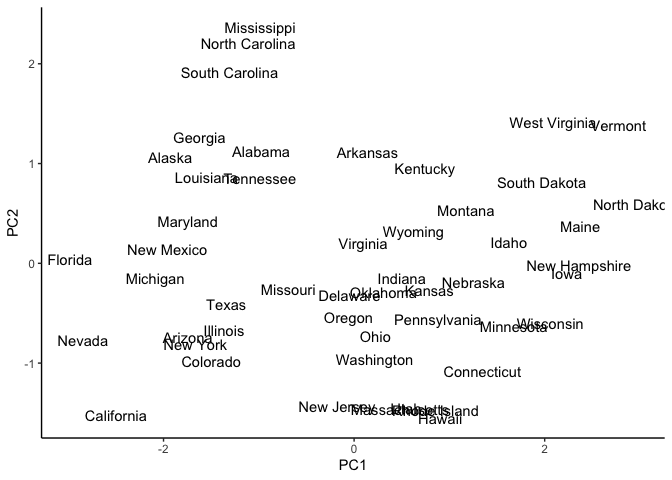We can already see possible clusters or grouping of states with similar statistics. Let’s start with an easy k value of 2. We initialize k and select for centroids. k = 2 centroids = sample.int(dim(USArrests_scaled), k) #randomly select k integers from 1 to the length of the data. centroid_points = USArrests_scaled[centroids,] %>% as.matrix() #use the selected integers as indices and select for them in the data frame. centroid_points  ## Murder Assault UrbanPop Rape ## Oregon -0.6630682 -0.1411127 0.1008652 0.8613783 ## Tennessee 1.2425641 0.2068693 -0.4518209 0.6051428  Next, we use a distance metric to compare the observation and the centroids. This will result in a matrix that gauges dissimilarity. Observations that are further apart from the centroid and less likely to be part of that cluster. Choice of distance metric will affect the formation of the clusters. Here we choose the Euclidean distance: $d_{euc}(x,y) =\sqrt{\Sigma_{i=1}^{n}(x_i-y_i)^2}$ •$n$is the number of observations •$y_i$is the value of the centroid dataPoints <- as.matrix( USArrests_scaled) dist_mat <- matrix(0, nrow = nrow(dataPoints), ncol = k) #initialize an empty matrix for (j in 1:k) { for (i in 1:nrow(dataPoints)) { dist_mat[i,j] = sqrt(sum((dataPoints[i,1:ncol(dataPoints)] - centroid_points[j,1:ncol(centroid_points)])^2)) } } head(dist_mat)  ## [,1] [,2] ## [1,] 2.370568 0.8407489 ## [2,] 2.699070 2.3362541 ## [3,] 2.000866 2.2989846 ## [4,] 1.847763 1.4254486 ## [5,] 2.657402 3.0119267 ## [6,] 1.533198 2.1972111  The cluster for each observation is chosen by the centroid with the smallest distance to the observation. We can use the which.min() function. cluster = factor(apply(dist_mat, 1, which.min)) #selects the column index with the smallest distance head(cluster)  ##  2 2 1 2 1 1 ## Levels: 1 2  Recall that we are minimizing the squared Euclidean distances between the observation and the assigned centroid. This is also the within-cluster sum of squares (WCSS). $W(C_k) =\Sigma_{x_i\in C_k}(x_i-\mu_k)^2$ We define a total within-cluster sum of squares (total_WCSS) which measures the compactness of the clustering. Minimizing this value results in tighter clusters. $totalWCSS =\Sigma^k_{k=1}W(C_k) =\Sigma^k_{k=1}\Sigma_{x_i\in C_k}(x_i-\mu_k)^2$ dist_mat_cluster <- list() for(i in 1:k){ dist_mat_cluster[[i]] <- dist_mat[which(cluster == i),i]^2 } within_cluster_ss <- unlist(lapply(dist_mat_cluster, sum)) cat('Within-cluster sum of squares:')  ## Within-cluster sum of squares:  within_cluster_ss  ##  133.74617 54.87014  total_WCSS = sum(within_cluster_ss) cat('\nTotal within-cluster sum of squares:', total_WCSS)  ## ## Total within-cluster sum of squares: 188.6163  Using the PCA graph, we can observe how our clusters look and where the initial centroids are located. pca_USArrests_df <- as.data.frame(pca_USArrests$x) %>%
dplyr::select(PC1, PC2) %>%
cbind(States = rownames(USArrests)) %>%
cbind(Clusters = cluster)

centroid_points_unscaled <- apply(centroid_points, 1,  function(x)
{ x * pca_USArrests$scale + pca_USArrests$center}) %>% t()
rownames(centroid_points_unscaled) <- c(1:k)

centroid_coord <- predict(pca_USArrests, centroid_points_unscaled) %>% as.data.frame() # adding the centroid coordinates

ggplot(pca_USArrests_df, aes(x = PC1, y = PC2,)) +
geom_text(aes(label = States, color = Clusters)) + # labeling the centroids
geom_point(data =  centroid_coord,
mapping =  aes(x = PC1, y = PC2,  color = rownames(centroid_coord)),
size = 3)  +
theme_classic()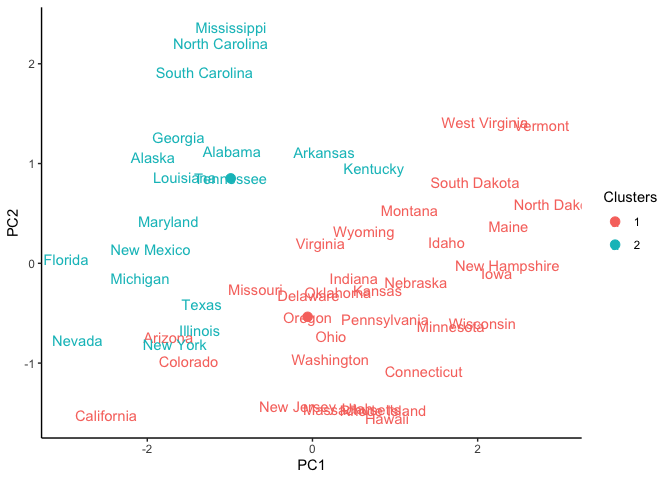As you can see, the clustering is not that great since we have only initialized the algorithm and had randomly selected k observations as centroids. The next step is to form new centroids and iteratively assign observations to clusters until a maximum number of iterations are reached or the observations no longer assigned to another cluster.

We can generate new centroid values by taking the mean of all values of each observations that are part of the cluster

new_centroid = USArrests_scaled %>%
as.data.frame() %>%
cbind(Clusters = cluster) %>%
group_by(Clusters) %>%
summarise_all(mean)
new_centroid

## # A tibble: 2 × 5
##   Clusters Murder Assault UrbanPop   Rape
##   <fct>     <dbl>   <dbl>    <dbl>  <dbl>
## 1 1        -0.629  -0.517   0.0620 -0.328
## 2 2         1.12    0.919  -0.110   0.584

centroid_points = new_centroid[,-1] %>% as.matrix()


## Creating a k-means function

Rather than repeating the code over and over, we can write a function that will do it for us.

k_means_ <- function(df, k, iters){
#initialize random centroids
centroids = sample.int(dim(df), k)
centroid_points = df[centroids,] %>% as.matrix()
dataPoints <- as.matrix(df)

#initialize WCSS
within_cluster_ss <- c()

for (i in 1:iters){
dist_mat <- matrix(0, nrow = nrow(dataPoints), ncol = k)
for (j in 1:k)
{
for (i in 1:nrow(dataPoints))
{
dist_mat[i,j] = sqrt(sum((dataPoints[i,1:ncol(dataPoints)] - centroid_points[j,1:ncol(centroid_points)])^2))
}
}

cluster = factor(apply(dist_mat, 1, which.min))
dist_mat_cluster <- list()
for(i in 1:k){
dist_mat_cluster[[i]] <- dist_mat[which(cluster == i),i]^2
}

within_cluster_ss_temp <- unlist(lapply(dist_mat_cluster, sum))
within_cluster_ss <- append(within_cluster_ss, within_cluster_ss_temp)

new_centroid = df %>%
as.data.frame() %>%
cbind(Clusters = cluster) %>%
group_by(Clusters) %>%
summarise_all(mean)
centroid_points = new_centroid[,-1] %>% as.matrix()
}
within_cluster_ss <- t(array(within_cluster_ss, dim = c(k, iters)))
return(list(Cluster = cluster,
WCSS = within_cluster_ss))
}


We use the same parameters as before and pass our variables into our new function k_means_().

iters = 10
k = 2
USArrests_scaled <- scale(USArrests)

k_means <- k_means_(USArrests_scaled, k, iters)
k_means

## $Cluster ##  2 2 2 1 2 2 1 1 2 2 1 1 2 1 1 1 1 2 1 2 1 2 1 2 2 1 1 2 1 1 2 2 2 1 1 1 1 1 ##  1 2 1 2 2 1 1 1 1 1 1 1 ## Levels: 1 2 ## ##$WCSS
##            [,1]     [,2]
##  [1,] 147.75655 82.14443
##  [2,]  64.39358 50.06885
##  [3,]  56.22017 46.82608
##  [4,]  56.11445 46.74796
##  [5,]  56.11445 46.74796
##  [6,]  56.11445 46.74796
##  [7,]  56.11445 46.74796
##  [8,]  56.11445 46.74796
##  [9,]  56.11445 46.74796
## [10,]  56.11445 46.74796


The total WCSS is minimized after reaching the maximum iterations.

df <- rowSums(k_means$WCSS) %>% as.data.frame() %>% cbind(iter = c(1:iters)) ggplot(df, aes(y =., x = iter)) + geom_line() + labs(x = 'Iteration', y = 'Total WCSS') + theme_classic()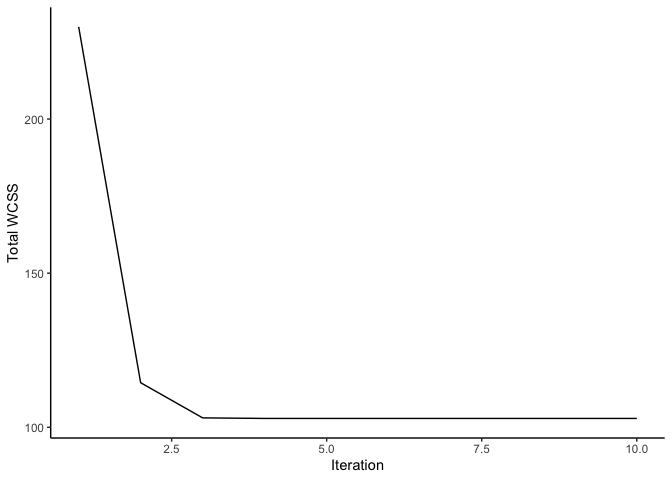Using the PCA graph, we can observe how our clusters look after reaching the maximum number of iterations. pca_USArrests_df <- as.data.frame(pca_USArrests$x) %>%
dplyr::select(PC1, PC2) %>%
cbind(States = rownames(USArrests)) %>%
cbind(Clusters = k_means$Cluster) ggplot(pca_USArrests_df, aes(x = PC1, y = PC2)) + geom_text(aes(label = States, color = Clusters)) + theme_classic()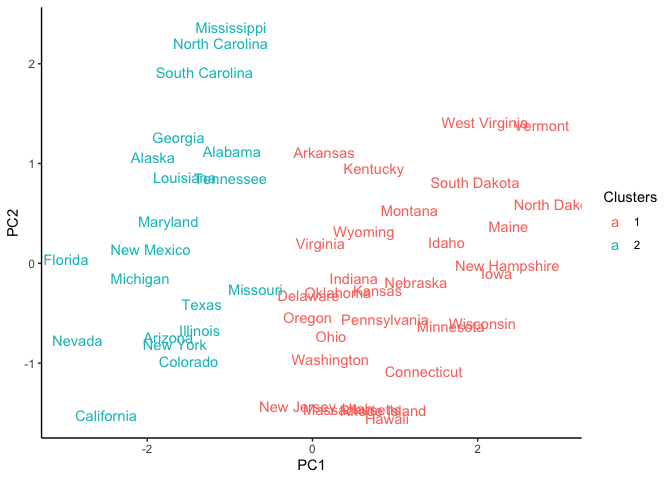We can also observe the clustering with a scatter plot of two features like UrbanPop and Murder. USArrests_df <- USArrests_scaled %>% as.data.frame() %>% cbind(States = rownames(USArrests)) %>% cbind(Clusters = k_means$Cluster)

ggplot(USArrests_df, aes(x = UrbanPop, y = Murder,)) +
geom_text(aes(label = States, color = Clusters)) +
theme_classic()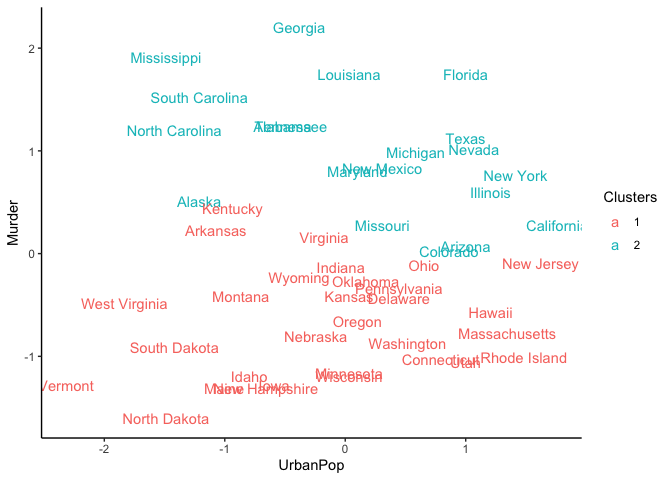## Determining the optimal number of clusters

Initially, we looked at 2 possible clusters. We can test out different numbers for k. Let’s repeat the process but with 2,3,4, and 5 clusters. The results are below:

k_means_test <- lapply(c(2:5), function(k) {k_means_(USArrests_scaled, k, iters)})
cluster_list <- lapply(k_means_test, function(x) x[])
names(cluster_list) <- paste('k =',c(2:5))

cluster_list_df <- do.call(cbind, cluster_list)

pca_USArrests_df <- as.data.frame(pca_USArrests\$x) %>%
dplyr::select(PC1, PC2) %>%
cbind(States = rownames(USArrests)) %>%
cbind(cluster_list_df) %>%
pivot_longer(cols = names(cluster_list))

ggplot(pca_USArrests_df, aes(x = PC1, y = PC2)) + geom_point(aes(shape = factor(value), color = factor(value))) + facet_wrap(~name) + labs(color  = "Cluster", shape = "Cluster")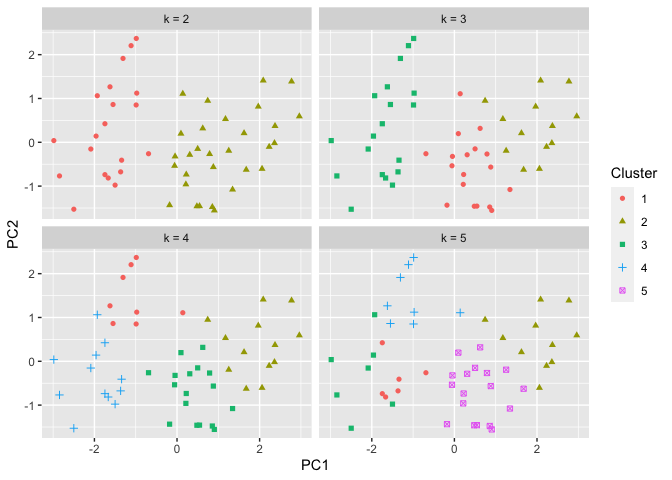Of course we can continue testing additional values of k. However, it may be more advantageous to determine the optimal k value based on the total within-cluster sum of squares. Recall that this value must be minimized to find the optimal cluster assignments. We can also use this to determine the optimal k.

1. Compute the k-means cluster for different values for k. For instance, we can vary k from 1 to 10.
2. For each value of k, the total within-cluster sum of squares.
3. Plot the the total within-cluster sum of squares against each value of k.
4. Determine the location of a bend in the plot. This typically indicates the optimal value for k.
k_means_test <- lapply(c(1:10), function(k) {k_means_(USArrests_scaled, k, iters)})
WCSS_list <- lapply(k_means_test, function(x) x[][iters,])
total_WCSS_list <- lapply(WCSS_list, sum)

df <- data.frame(Y = unlist(total_WCSS_list), X = c(1:10))

ggplot(df, aes(x = X, y = Y)) +
geom_line() +
geom_point() +
labs(x = 'k clusters', y = 'Total WCSS') +
scale_x_continuous(breaks = c(1:10)) +
theme_classic()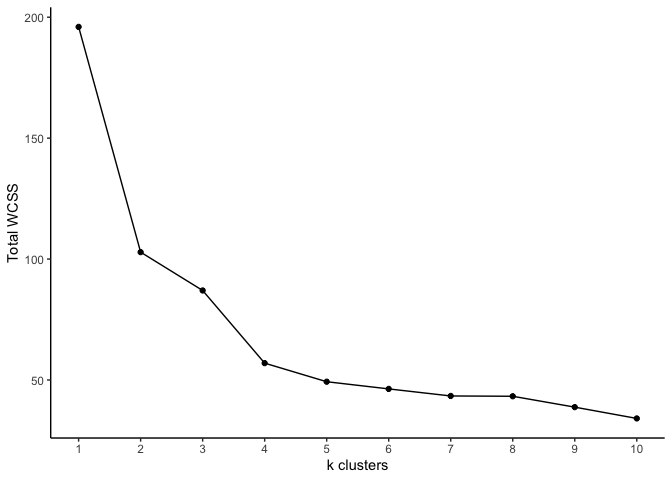The optimal k` look to be either 4 or 5. As you can see, k-means clustering is simple and quick. One caveat is choosing the number of clusters. Another is the random initialization of centroids. This could slow down the algorithm in very large data sets. One possible improvement is to generate different initial centroids and select the set that has the smallest total within-cluster sum of squares.